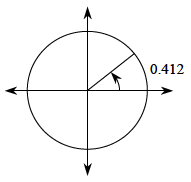### Home > PC > Chapter 10 > Lesson 10.1.1 > Problem10-9

10-9.

Solve the trigonometric equations for the variable in the given interval.

1. $7 \sin x − 9 = 2 \sin x − 7$ $[ 0, 2\pi]$

Solve this equation using the same steps as you would to solve the equation
$7x − 9 = 2x − 7$.

How do you 'undo' the sine function?

You will need to use the $\sin^{−1}$ key on your calculator. Remember that your calculator will only give one answer.

$x = 0.412$

Draw a unit cirlce to find the 2nd answer. Recall that $π ≈ 3.14$.1. $4 \sin^{2} α = 3$ $[0, 2\pi]$

Solve this equation using the same steps as you wold to solve the equation
$4x^2 = 3$.

Don't forget the ± sign.

You do not need your calculator and should find 4 solutions.